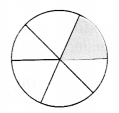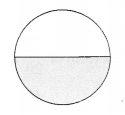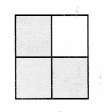# CBSEtips.in

## Sunday, 28 February 2021

### CBSE Class 6 Maths - MCQ and Online Tests- Unit 7 - Fractions

#### CBSE Class 6 Maths – MCQ and Online Tests – Unit 7 – Fractions

Every year CBSE students attend Annual Assessment exams for 6,7,8,9,11th standards. These exams are very competitive to all the students. So our website provides online tests for all the 6,7,8,9,11th standards’ subjects. These tests are also very effective and useful for those who preparing for any competitive exams like Olympiad etc. It can boost their preparation level and confidence level by attempting these chapter wise online tests.

These online tests are based on latest CBSE syllabus. While attempting these, our students can identify their weak lessons and continuously practice those lessons for attaining high marks. It also helps to revise the NCERT textbooks thoroughly.#### CBSE Class 6 Maths – MCQ and Online Tests – Unit 7 – Fractions

Question 1.
What fraction of an hour is 30 minutes?
(a) $$\frac { 1 }{ 4 }$$
(b) $$\frac { 1 }{ 2 }$$
(c) $$\frac { 1 }{ 3 }$$
(d) $$\frac { 1 }{ 5 }$$

Question 2.
Which of the following is a proper fraction?
(a) $$\frac { 0 }{ 1 }$$
(b) $$\frac { 5 }{ 2 }$$
(c) $$\frac { 7 }{ 4 }$$
(d) $$\frac { 11 }{ 3 }$$

Question 3.
What fraction of a day is 12 hours?
(a) $$\frac { 1 }{ 2 }$$
(b) $$\frac { 1 }{ 4 }$$
(c) $$\frac { 1 }{ 3 }$$
(d) $$\frac { 1 }{ 6 }$$

Question 4.
Which of the following is a proper fraction?
(a) $$\frac { 1 }{ 2 }$$
(b) $$\frac { 5 }{ 4 }$$
(c) $$\frac { 3 }{ 2 }$$
(d) $$\frac { 9 }{ 2 }$$

Question 5.
The fraction representing the shaded portion is(a) $$\frac { 1 }{ 4 }$$
(b) $$\frac { 3 }{ 4 }$$
(c) $$\frac { 1 }{ 2 }$$
(d) $$\frac { 1 }{ 8 }$$

Question 6.
Which of the following is a proper fraction whose numerator is 1 and denominator is 3?
(a) $$\frac { 1 }{ 3 }$$
(b) $$\frac { 1 }{ 6 }$$
(c) $$\frac { 1 }{ 9 }$$
(d) $$\frac { 1 }{ 12 }$$

Question 7.
Which of the following fractions is not equivalent to $$\frac { 1 }{ 3 }$$ ?
(a) $$\frac { 5 }{ 15 }$$
(b) $$\frac { 6 }{ 18 }$$
(c) $$\frac { 4 }{ 12 }$$
(d) $$\frac { 7 }{ 20 }$$

Question 8.
Express $$\frac { 9 }{ 4 }$$ as a mixed fraction.
(a) 2 $$\frac { 1 }{ 4 }$$
(b) 3 $$\frac { 1 }{ 4 }$$
(c) 4 $$\frac { 1 }{ 4 }$$
(d) 5 $$\frac { 1 }{ 4 }$$

Question 9.
Which of the following fractions is equivalent to $$\frac { 3 }{ 4 }$$?
(a) $$\frac { 6 }{ 11 }$$
(b) $$\frac { 9 }{ 10 }$$
(c) $$\frac { 15 }{ 20 }$$
(d) $$\frac { 21 }{ 25 }$$

Question 10.
Which of the following is an improper fraction?
(a) $$\frac { 2 }{ 3 }$$
(b) $$\frac { 3 }{ 4 }$$
(c) $$\frac { 4 }{ 5 }$$
(d) $$\frac { 5 }{ 4 }$$

Question 11.
The fraction representing the shaded portion is(a) $$\frac { 1 }{ 2 }$$
(b) $$\frac { 1 }{ 4 }$$
(c) $$\frac { 1 }{ 3 }$$
(d) $$\frac { 1 }{ 6 }$$

Question 12.
Which of the following is an improper fraction?
(a) $$\frac { 1 }{ 4 }$$
(b) $$\frac { 3 }{ 8 }$$
(c) $$\frac { 11 }{ 3 }$$
(d) $$\frac { 2 }{ 5 }$$

Question 13.
Express $$\frac { 5 }{ 2 }$$ as a mixed fraction.
(a) 1 $$\frac { 1 }{ 2 }$$
(b) 2 $$\frac { 1 }{ 2 }$$
(c) 3 $$\frac { 1 }{ 2 }$$
(d) 4 $$\frac { 1 }{ 2 }$$

Question 14.
Express the mixed fraction 1$$\frac { 1 }{ 2 }$$ as an improper fraction.
(a) $$\frac { 2 }{ 3 }$$
(b) $$\frac { 3 }{ 2 }$$
(c) $$\frac { 3 }{ 4 }$$
(d) none of these

Question 15.
The fraction representing the shaded portion is(a) $$\frac { 1 }{ 4 }$$
(b) $$\frac { 1 }{ 2 }$$
(c) $$\frac { 1 }{ 3 }$$
(d) $$\frac { 1 }{ 8 }$$

Question 16.
The fraction representing the shaded portion is(a) $$\frac { 1 }{ 4 }$$
(b) $$\frac { 1 }{ 2 }$$
(c) $$\frac { 3 }{ 4 }$$
(d) none of these

Question 17.
The equivalent fraction of $$\frac { 2 }{ 5 }$$ with numerator 4 is
(a) $$\frac { 4 }{ 10 }$$
(b) $$\frac { 4 }{ 12 }$$
(c) $$\frac { 4 }{ 16 }$$
(d) $$\frac { 4 }{ 20 }$$

Question 18.
The fraction representing the shaded portion is(a) $$\frac { 1 }{ 4 }$$
(b) $$\frac { 1 }{ 2 }$$
(c) $$\frac { 1 }{ 8 }$$
(d) none of these

Question 19.
Express the mixed fraction 2 $$\frac { 3 }{ 4 }$$ as an improper fraction.
(a) $$\frac { 11 }{ 4 }$$
(b) $$\frac { 4 }{ 11 }$$
(c) $$\frac { 9 }{ 11 }$$
(d) $$\frac { 7 }{ 11 }$$

Question 20.
What fraction of ₹ 1 is 50 paise?
(a) $$\frac { 1 }{ 2 }$$
(b) $$\frac { 1 }{ 4 }$$
(c) $$\frac { 1 }{ 8 }$$
(d) $$\frac { 1 }{ 10 }$$

Question 21.
The simplest form of $$\frac { 12 }{ 20 }$$ is
(a) $$\frac { 1 }{ 5 }$$
(b) $$\frac { 2 }{ 5 }$$
(c) $$\frac { 3 }{ 5 }$$
(d) $$\frac { 4 }{ 5 }$$

Question 22.
The simplest form of $$\frac { 45 }{ 20 }$$ is
(a) $$\frac { 9 }{ 4 }$$
(b) $$\frac { 4 }{ 9 }$$
(c) $$\frac { 9 }{ 8 }$$
(d) $$\frac { 2 }{ 9 }$$

Question 23.
The equivalent fraction of $$\frac { 20 }{ 36 }$$ with denominator 9 is
(a) $$\frac { 4 }{ 9 }$$
(b) $$\frac { 5 }{ 9 }$$
(c) $$\frac { 7 }{ 9 }$$
(d) $$\frac { 8 }{ 9 }$$

Question 24.
What fraction of ₹ 1 is 25 paise?
(a) $$\frac { 1 }{ 2 }$$
(b) $$\frac { 1 }{ 4 }$$
(c) $$\frac { 1 }{ 8 }$$
(d) $$\frac { 1 }{ 10 }$$

Question 25.
Which of the following pairs of fractions are equivalent?
(a) $$\frac { 1 }{ 2 }$$ , $$\frac { 2 }{ 4 }$$
(b) $$\frac { 1 }{ 3 }$$ , $$\frac { 3 }{ 7 }$$
(c) $$\frac { 2 }{ 5 }$$ , $$\frac { 4 }{ 9 }$$
(d) $$\frac { 4 }{ 7 }$$ , $$\frac { 8 }{ 13 }$$